# Quantum Physics

If quantum cosmologists can reconcile quantum mechanics with Einstein's Theory of General Relativity, we may be in for a wild ride, as scientists will finally have a single theory with the potential to describe all aspects of time and space in the universe. In short, we will be on our way to knowing the unknowable. Quite exciting, to say the least. At the heart of quantum theory lies such ideas as the particle nature of waves, the wave-like properties of particles, the quantized energy levels of atoms, and the Heisenberg Uncertainty Principle. It is from these fundamental assumptions about the natural world that we may be able to unlock its secrets through the development of a unified field theory. A good course in quantum physics will take students through the following areas:

• The wave function
• Time-independent Schrodinger equation
• Formalism
• Quantum mechanics in three dimensions
• Identical particles
• Time-independent perturbation theory
• The variational principle
• The WKB approximation
• Time-dependent perturbation theory
• Scattering

There are plenty of books on quantum physics to be found on Google and Amazon.com. There is also a nice collection of books and journals devoted to this area which students can find at the Springer website.

## The variational principle

If a quantum system has a Hamiltonian independent of time  and one chooses a normalized “well behaved” function  (i.e. a continuous function for which the first and second derivatives exist) then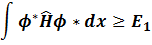where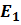is the lowest energy eigenvalue of the Hamiltonian (bound energy).

In other words, if we chose a random test function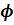the bounded energy will be minimum of the expression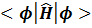Question 1

Consider a one-dimensional system of a particle with a potential energy: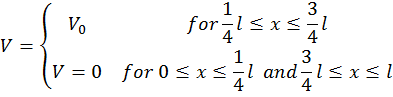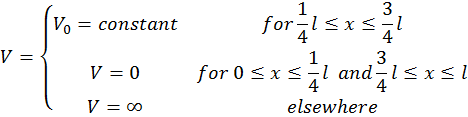Make a graph of V vs X. Use the test function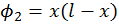to determine the energy of the ground level. Note that there is no variational parameter.  Note that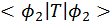is given by the equation (why?):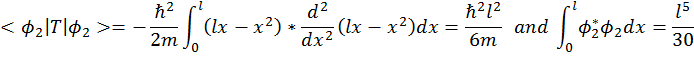Solution: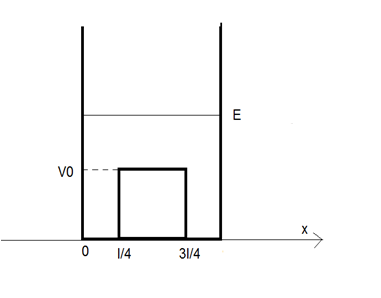If you consider a test function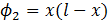having no variational parameter (that is having no constant coefficients inside) then the eigenvalue of the bound level can be approximated by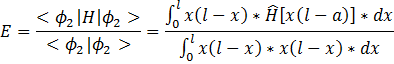Since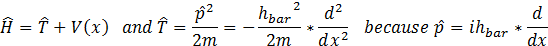We can write: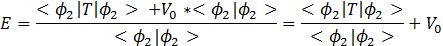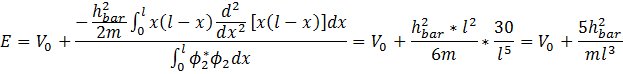since we have: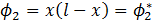Question 2

Consider you have a test function: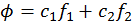where f1 and f2 are real functions. Find the energy of the bound level in terms of the integrals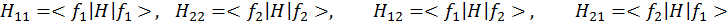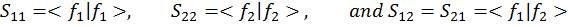For the special case when: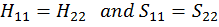find also the rapport of the coefficients: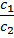Solution:

If you have a test function of the type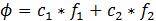Then the energy of the bound level is given by (like above):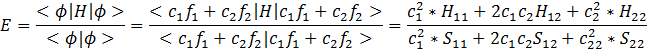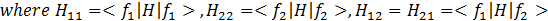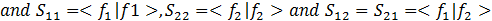Which for: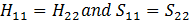gives the rapport of coefficients: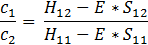To have a solution of the above system of two equations one needs to have the characteristic determinant = 0. In other words the condition for existence of E states is: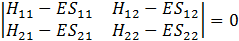or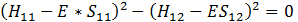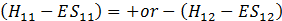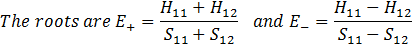#### To fulfill our tutoring mission of online education, our college homework help and online tutoring centers are standing by 24/7, ready to assist college students who need homework help with all aspects of quantum physics. Our physics tutors can help with all your projects, large or small, and we challenge you to find better online quantum physics tutoring anywhere.

Get College Homework Help.

Are you sure you don't want to upload any files?

Fast tutor response requires as much info as possible.

We couldn't find that subject.
Please select the best match from the list below.
For faster response, you may skip assigning directly to a tutor to receive the first tutor available.
That tutor may not be available for several hours. Please try another tutor if you're in a hurry.

We'll send you an email right away. If it's not in your inbox, check your spam folder.

• 1
• 2
• 3

## Latest News